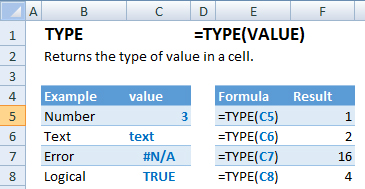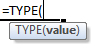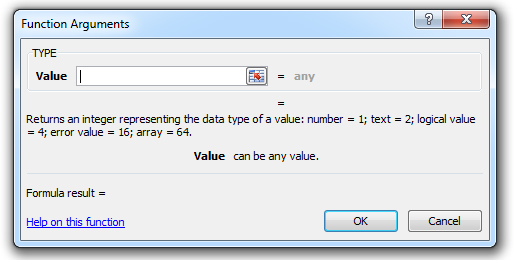### TYPE Function Examples – Excel, VBA, & Google Sheets

This Excel Tutorial demonstrates how to use the Excel TYPE Function in Excel to get the type of information containined in a cell, with formula examples.

## TYPE Function Description:

The TYPE Function Returns an integer representing the type of value within the cell.

## Formula Examples:Example Formula Result
Number =TYPE(C5) 1
Text =TYPE(C6) 2
Error =TYPE(C7) 16
Logical =TYPE(C8) 4
AutoMacro - VBA Code Generator

## Syntax and Arguments:

The Syntax for the TYPE Formula is:

`=TYPE(VALUE)`

### Function Arguments ( Inputs ):

value – The test value

## Additional Notes

Integer results from the TYPE Function: <>

## TYPE Examples in VBA

You can also use the TYPE function in VBA. Type:
`application.worksheetfunction.type(value)`
For the function arguments (value, etc.), you can either enter them directly into the function, or define variables to use instead.

Return to the List of all Functions in Excel

## How to use the TYPE Function in Excel:

To use the AND Excel Worksheet Function, type the following into a cell:
`=AND(`
After entering it in the cell, notice how the AND formula inputs appear below the cell:You will need to enter these inputs into the function. The function inputs are covered in more detail in the next section. However, if you ever need more help with the function, after typing “=TYPE(” into a cell, without leaving the cell, use the shortcut CTRL + A (A for Arguments) to open the “Insert Function Dialog Box” for detailed instructions:For more information about the TYPE Formula visit the
Microsoft Website.

## Excel Practice Worksheet

Practice Excel functions and formulas with our 100% free practice worksheets!

• Automatically Graded Exercises
• Learn Excel, Inside Excel!